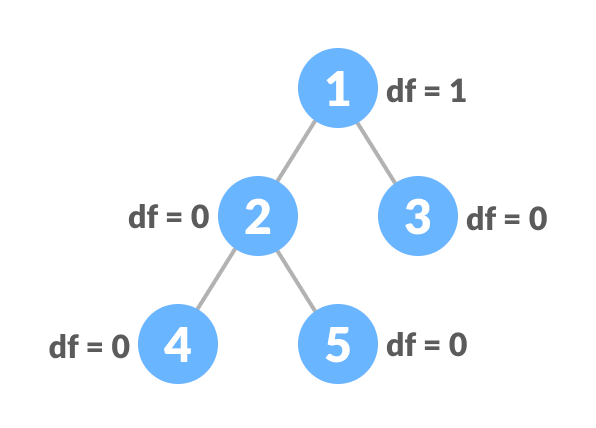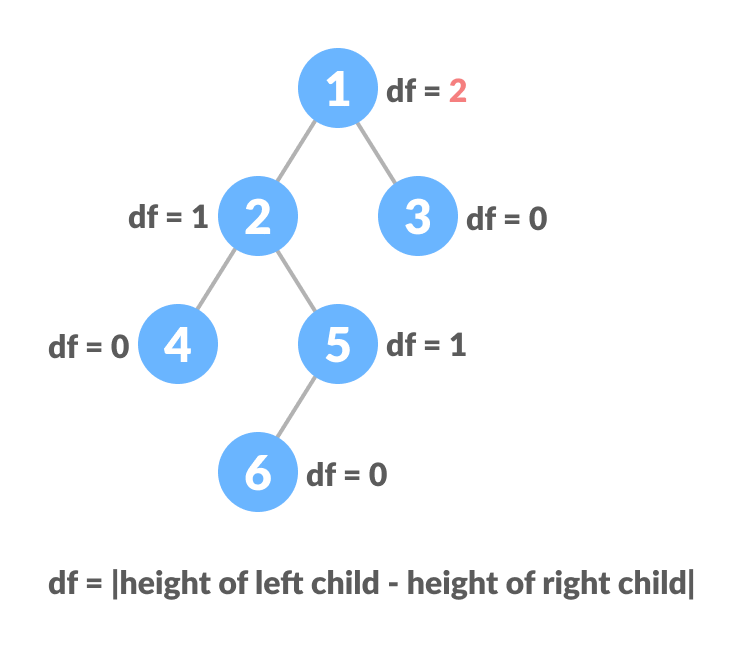Hits: 3

# Balanced Binary Tree

#### In this tutorial, you will learn about a balanced binary tree and its different types. Also, you will find working examples of a balanced binary tree in Python.

A balanced binary tree, also referred to as a height-balanced binary tree, is defined as a binary tree in which the height of the left and right subtree of any node differ by not more than 1.

To learn more about the height of a tree/node, visit Tree Data Structure.Following are the conditions for a height-balanced binary tree:

1. difference between the left and the right subtree for any node is not more than one
2. the left subtree is balanced
3. the right subtree is balancedBalanced Binary Tree with depth at each levelUnbalanced Binary Tree with depth at each level

## Python Examples

The following code is for checking whether a tree is height-balanced.

``````/* Checking if a binary tree is CalculateHeight balanced in Python */

/* CreateNode creation */
class CreateNode:

def __init__(self, item):
self.item = item
self.left = self.right = None

/* Calculate height */
class CalculateHeight:
def __init__(self):
self.CalculateHeight = 0

/* Check height balance */
def is_height_balanced(root, CalculateHeight):

left_height = CalculateHeight()
right_height = CalculateHeight()

if root is None:
return True

l = is_height_balanced(root.left, left_height)
r = is_height_balanced(root.right, right_height)

CalculateHeight.CalculateHeight = max(
left_height.CalculateHeight, right_height.CalculateHeight) + 1

if abs(left_height.CalculateHeight - right_height.CalculateHeight) <= 1:
return l and r

return False

CalculateHeight = CalculateHeight()

root = CreateNode(1)
root.left = CreateNode(2)
root.right = CreateNode(3)
root.left.left = CreateNode(4)
root.left.right = CreateNode(5)

if is_height_balanced(root, CalculateHeight):
print('The tree is balanced')
else:
print('The tree is not balanced')
``````

# Special 95% discount

## 2000+ Applied Machine Learning & Data Science Recipes

### Portfolio Projects for Aspiring Data Scientists: Tabular Text & Image Data Analytics as well as Time Series Forecasting in Python & R## Two Machine Learning Fields

There are two sides to machine learning:

• Practical Machine Learning:This is about querying databases, cleaning data, writing scripts to transform data and gluing algorithm and libraries together and writing custom code to squeeze reliable answers from data to satisfy difficult and ill defined questions. It’s the mess of reality.
• Theoretical Machine Learning: This is about math and abstraction and idealized scenarios and limits and beauty and informing what is possible. It is a whole lot neater and cleaner and removed from the mess of reality.

Data Science Resources: Data Science Recipes and Applied Machine Learning Recipes

Introduction to Applied Machine Learning & Data Science for Beginners, Business Analysts, Students, Researchers and Freelancers with Python & R Codes @ Western Australian Center for Applied Machine Learning & Data Science (WACAMLDS) !!!

Latest end-to-end Learn by Coding Recipes in Project-Based Learning:

Applied Statistics with R for Beginners and Business Professionals

Data Science and Machine Learning Projects in Python: Tabular Data Analytics

Data Science and Machine Learning Projects in R: Tabular Data Analytics

Python Machine Learning & Data Science Recipes: Learn by Coding

R Machine Learning & Data Science Recipes: Learn by Coding

Comparing Different Machine Learning Algorithms in Python for Classification (FREE)

`Disclaimer: The information and code presented within this recipe/tutorial is only for educational and coaching purposes for beginners and developers. Anyone can practice and apply the recipe/tutorial presented here, but the reader is taking full responsibility for his/her actions. The author (content curator) of this recipe (code / program) has made every effort to ensure the accuracy of the information was correct at time of publication. The author (content curator) does not assume and hereby disclaims any liability to any party for any loss, damage, or disruption caused by errors or omissions, whether such errors or omissions result from accident, negligence, or any other cause. The information presented here could also be found in public knowledge domains.  `